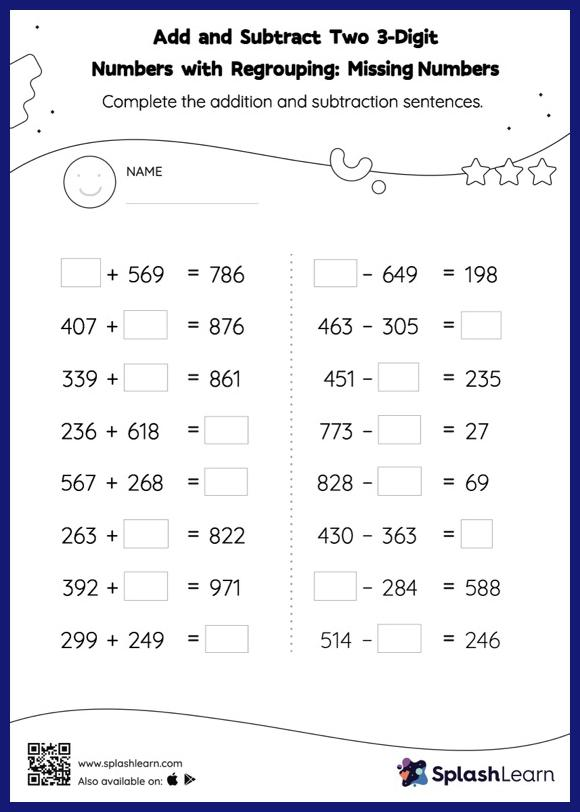# Add and Subtract Two 3-Digit Numbers with Regrouping: Missing Numbers Worksheet

Home > Add and Subtract Two 3-Digit Numbers with Regrouping: Missing NumbersStudents demonstrate their problem-solving ability as they work on this add and subtract two 3-digit numbers with regrouping worksheet. In this worksheet, students must find the missing number using the relationship between addition and subtraction. To solve add and subtract two 3-digit numbers with regrouping worksheet, they must also regroup the numbers.